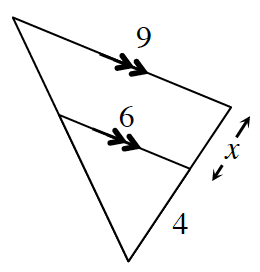### Home > GC > Chapter 12 > Lesson 12.1.1 > Problem12-11

12-11.

Multiple Choice: In the diagram at right, the value of $x$ is:

1. $1$

1. $2$

1. $3$

1. $4$

1. none of theseThe diagram contains similar triangles. Use this fact to set up a proportion.

$\frac{6}{9} = \frac{4}{4+x}$

b. $2$